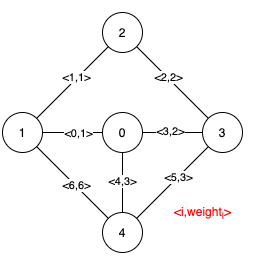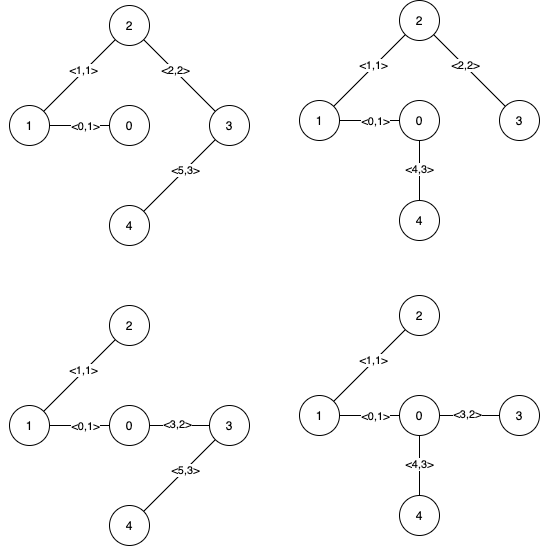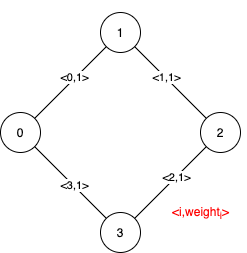# GeetCode Hub

Given a weighted undirected connected graph with `n` vertices numbered from `0` to `n - 1`, and an array `edges` where `edges[i] = [ai, bi, weighti]` represents a bidirectional and weighted edge between nodes `ai` and `bi`. A minimum spanning tree (MST) is a subset of the graph's edges that connects all vertices without cycles and with the minimum possible total edge weight.

Find all the critical and pseudo-critical edges in the given graph's minimum spanning tree (MST). An MST edge whose deletion from the graph would cause the MST weight to increase is called a critical edge. On the other hand, a pseudo-critical edge is that which can appear in some MSTs but not all.

Note that you can return the indices of the edges in any order.

Example 1:```Input: n = 5, edges = [[0,1,1],[1,2,1],[2,3,2],[0,3,2],[0,4,3],[3,4,3],[1,4,6]]
Output: [[0,1],[2,3,4,5]]
Explanation: The figure above describes the graph.
The following figure shows all the possible MSTs:Notice that the two edges 0 and 1 appear in all MSTs, therefore they are critical edges, so we return them in the first list of the output.
The edges 2, 3, 4, and 5 are only part of some MSTs, therefore they are considered pseudo-critical edges. We add them to the second list of the output.
```

Example 2:```Input: n = 4, edges = [[0,1,1],[1,2,1],[2,3,1],[0,3,1]]
Output: [[],[0,1,2,3]]
Explanation: We can observe that since all 4 edges have equal weight, choosing any 3 edges from the given 4 will yield an MST. Therefore all 4 edges are pseudo-critical.
```

Constraints:

• `2 <= n <= 100`
• `1 <= edges.length <= min(200, n * (n - 1) / 2)`
• `edges[i].length == 3`
• `0 <= ai < bi < n`
• `1 <= weighti <= 1000`
• All pairs `(ai, bi)` are distinct.

class Solution { public List<List<Integer>> findCriticalAndPseudoCriticalEdges(int n, int[][] edges) { } }